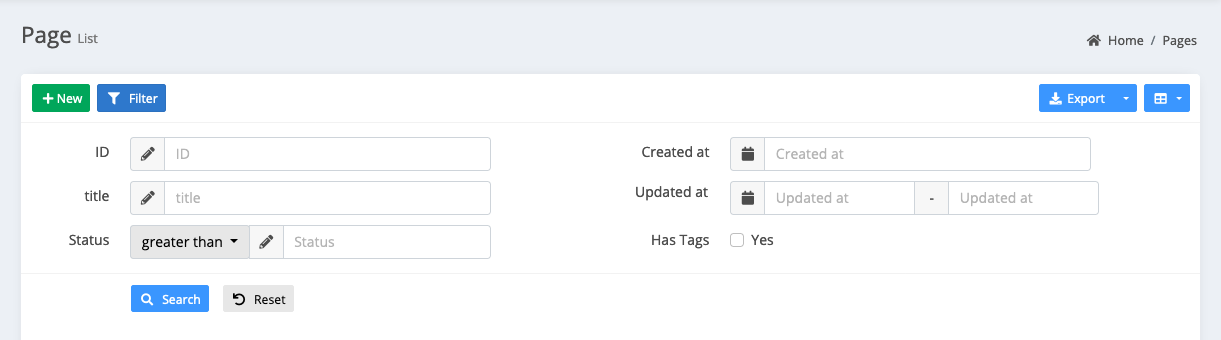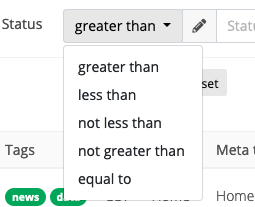# Model grid filters

## Introduction

`model-grid`Provides a set of data filters:

``````\$grid->filter(function(\$filter){

// Remove the default id filter
\$filter->disableIdFilter();

\$filter->like('name', 'name');

});``````

### Show Filter Open

Adjust the style of the filter query panel, from the original pop-up modal to the embedded table header, click the filter button to expand the display, the default is not expanded, you can let it expand by default in the following way:

``````// Operate on the `\$grid` instance
\$grid->expandFilter();

// Or manipulate the `\$filter` instance in the filter callback
\$filter->expand();``````

### Define the scope of the query

You can define your most commonly used query as a query scope, which will appear in the drop-down menu of the filter button. Here are a few examples:

``````\$filter->scope('male', 'Male')->where('gender', 'm');

// Multiple conditional query
\$filter->scope('new', 'Recently modified')
->whereDate('created_at', date('Y-m-d'))
->orWhere('updated_at', date('Y-m-d'));

// Relationship query
});

\$filter->scope('trashed', 'Soft deleted data')->onlyTrashed();``````

The first parameter of the `scope` method is the key of the query. It will appear in the url parameter. The second parameter is the label of the drop-down menu item. If not filled, the first parameter will be displayed as the label.

You can call any `eloquent` query condition after the `scope` method. The effect is referenced Demo

## Filter types

Currently supported filter types are the following:

### Equal

`sql: ... WHERE`column`= ""\$input""`

``\$filter->equal('column', \$label);``

### Not equal

`sql: ... WHERE`column`!= ""\$input""`

``\$filter->notEqual('column', \$label);``

### Like

`sql: ... WHERE`column`LIKE "%"\$input"%"`

``\$filter->like('column', \$label);``

### Ilike

`sql: ... WHERE`column`ILIKE "%"\$input"%"`

``\$filter->ilike('column', \$label);``

### contains

Equal to like query

``\$filter->contains('title');``

### starts with

Query title field data starting with input

``\$filter->startsWith('title');``

### starts with

Query title field data ending with input

``\$filter->endsWith('title');``

### Greater then

`sql: ... WHERE`column`> "\$input"`

``\$filter->gt('column', \$label);``

### Less than

`sql: ... WHERE`column`< "\$input"`

``\$filter->lt('column', \$label);``

### Between

`sql: ... WHERE`column`BETWEEN "\$start" AND "\$end"`

``````\$filter->between('column', \$label);

// set datetime field type
\$filter->between('column', \$label)->datetime();

// set time field type
\$filter->between('column', \$label)->time();``````

### In

`sql: ... WHERE`column`in (...\$inputs)`

``\$filter->in('column', \$label)->multipleSelect(['key' => 'value']);``

### NotIn

`sql: ... WHERE`column`not in (...\$inputs)`

``\$filter->notIn('column', \$label)->multipleSelect(['key' => 'value']);``

### Date

`sql: ... WHERE DATE(`column`) = "\$input"`

``\$filter->date('column', \$label);``

### Day

`sql: ... WHERE DAY(`column`) = "\$input"`

``\$filter->day('column', \$label);``

### Month

`sql: ... WHERE MONTH(`column`) = "\$input"`

``\$filter->month('column', \$label);``

### year

`sql: ... WHERE YEAR(`column`) = "\$input"`

``\$filter->year('column', \$label);``

### Where

You can use `where` to build more complex query filtering

`sql: ... WHERE`title`LIKE "%\$input" OR`content`LIKE "%\$input"`

``````\$filter->where(function (\$query) {

\$query->where('title', 'like', "%{\$this->input}%")
->orWhere('content', 'like', "%{\$this->input}%");

}, 'Text');``````

`sql: ... WHERE`rate`>= 6 AND`created_at`= {\$input}`:

``````\$filter->where(function (\$query) {

\$query->whereRaw("`rate` >= 6 AND `created_at` = {\$this->input}");

}, 'Text');``````

Relationship query, query the corresponding relationship `profile` field:

``````\$filter->where(function (\$query) {

\$query->whereHas('profile', function (\$query) {
});

## Field type

The default field type is text input, set placeholder for text input:

``\$filter->equal('column')->placeholder('Please input...');``

You can also restrict the user input format by using some of the following methods:

``````\$filter->equal('column')->url();

\$filter->equal('column')->email();

\$filter->equal('column')->integer();

\$filter->equal('column')->ip();

\$filter->equal('column')->mac();

\$filter->equal('column')->mobile();

\$filter->equal('column')->decimal(\$options = []);

\$filter->equal('column')->currency(\$options = []);

\$filter->equal('column')->percentage(\$options = []);

\$filter->equal('column')->inputmask(\$options = [], \$icon = 'pencil');``````

### Select

``````\$filter->equal('column')->select(['key' => 'value'...]);

// Or from the api to obtain data, api format reference model-form `select` component
\$filter->equal('column')->select('api/users');``````

### multipleSelect

Generally used in conjunction with `in` and`notIn` need to query the array of two types of inquiries can also be used in the `type` type of query:

``````\$filter->in('column')->multipleSelect(['key' => 'value'...]);

// // Or from the api to obtain data, api format reference model-form `multipleSelect` component
\$filter->in('column')->multipleSelect('api/users');``````

The more common scenario is the selection of categories

``````\$filter->equal('released')->radio([
''   => 'All',
0    => 'Unreleased',
1    => 'Released',
]);``````

### checkbox

The more common scene is do the scope query with `whereIn`:

``````\$filter->in('gender')->checkbox([
'm'    => 'Male',
'f'    => 'Female',
]);``````

### Datetime

Use date and time components,`\$options` parameter and value reference flatpickr

``````\$filter->equal('column')->datetime(\$options);

// `date()` equals to `datetime(['format' => 'YYYY-MM-DD'])`
\$filter->equal('column')->date();

// `time()` equals to `datetime(['format' => 'HH:mm:ss'])`
\$filter->equal('column')->time();``````

below functions just a regular text field

``````// `day()` equals to `datetime(['format' => 'DD'])`
\$filter->equal('column')->day();

// `month()` equals to `datetime(['format' => 'MM'])`
\$filter->equal('column')->month();

// `year()` equals to `datetime(['format' => 'YYYY'])`
\$filter->equal('column')->year();
``````

## Complex query filter

You can use the `\$this->input` to trigger complex custom queries:

``````\$filter->where(function (\$query) {
switch (\$this->input) {
case 'yes':
// custom complex query if the 'yes' option is selected
\$query->has('somerelationship');
break;
case 'no':
\$query->doesntHave('somerelationship');
break;
}
}, 'Label of the field', 'name_for_url_shortcut')->radio([
'' => 'All',
'yes' => 'Only with relationship',
'no' => 'Only without relationship',
]);
``````

### Multi-column layout

If there are too many filters, the page will be pulled long, which will affect the look and feel of the page. This version will support the multi-column layout of the filter. For example, 6 filters are displayed in two columns.

``````    \$grid->filter(function (\$filter) {
\$filter->expand();

\$filter->column(1/2, function (\$filter) {
\$filter->like('title', 'title');
\$filter->group('status', function (\$group) {
\$group->gt('greater than');
\$group->lt('less than');
\$group->nlt('not less than');
\$group->ngt('not greater than');
\$group->equal('equal to');
});
});

\$filter->column(1/2, function (\$filter) {
\$filter->equal('created_at')->datetime();
\$filter->between('updated_at')->datetime();
\$filter->where(function (\$query) {
\$query->whereHas('tags', function (\$query) {
\$query->whereNotNull('tag');
});
}, 'Has Tags')->checkbox([
1    => 'Yes'

]);
});
});``````

By default, there will be a filter for the primary key field in the first column, and all three filters for the left and right will have a total of 6 filters.

The first parameter of the `column` method sets the column width, which can be set to the scale `1/2` or `0.5`, or the width of the grid column of the bootstrap is `6`. If it is three columns, it can be set to `1/3.`or `4`### Filter group

Sometimes you need to set multiple filtering methods for the same field, you can do it in the following way.

``````\$filter->group('rate', function (\$group) {
\$group->gt('greater than');
\$group->lt('less than');
\$group->nlt('not less than');
\$group->ngt('not greater than');
\$group->equal('equal to');
});``````

There are several ways to call

``````// equals
\$group->equal();

// not equal to
\$group->notEqual();

// more than the
\$group->gt();

// less than
\$group->lt();

// greater or equal to
\$group->nlt();

// less than or equal to
\$group->ngt();

// match
\$group->match();

// Complex conditions
\$group->where();

// like query
\$group->like();

// like query
\$group->contains();

// ilike query
\$group->ilike();